Next: Inverse filter example Up: Model fitting by least Previous: Model fitting by least

# MULTIVARIATE LEAST SQUARES

As described at the beginning of chapter, signals and images will be specified here by numbers packed into abstract vectors. We consider first a hypothetical application with one data vector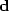and two fitting vectorsand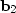.Each fitting vector is also known as a regressor." Our first task is to try to approximate the data vectorby a scaled combination of the two regressor vectors. The scale factors x1 and x2 should be chosen so that the model matches the data, i.e.,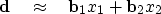(1)
For example, if I print the characters'' and'' on top of each other, I get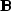,'' which looks something like an image of the letter.'' This is analogous to.More realistically,could contain a sawtooth function of time, andandcould be sinusoids. Still more realistically,could be an observed 2-D wave field, andandcould be theoretical data in two parts, where the contribution of each part is to be learned by fitting. (One part could be primary reflections and the other multiple reflections.)

Notice that we could take the partial derivative of the data in (1) with respect to an unknown, say x1, and the result is the regressor.

 The partial derivative of all data with respect to any model parameter gives a regressor. A regressor is a column in the partial-derivative matrix.

Equation (1) is often expressed in the more compact mathematical matrix notation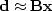,but in our derivation here we will keep track of each component explicitly and use mathematical matrix notation to summarize the final result. Fitting the datato its two theoretical components can be expressed as minimizing the length of the residual vector, where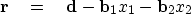(2)
So we construct a sum of squares (also called a quadratic form") of the components of the residual vector by using a dot product:(3) (4)
The gradient of Q(x1,x2)/2 is defined by its two components:(5) (6)
Setting these derivatives to zero and using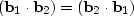etc., we get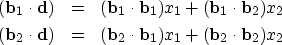(7) (8)
which two equations we can use to solve for the two unknowns x1 and x2. Writing this expression in matrix notation, we have(9)
It is customary to use matrix notation without dot products. For this we need some additional definitions. To clarify these definitions, I choose the number of components in the vectors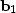,, andto be three. Thus I can explicitly write a matrix boldB in full as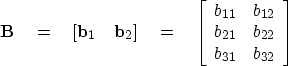(10)
Likewise, the transposed matrix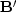is defined by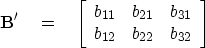(11)
The matrix in equation (9) contains dot products. Matrix multiplication is an abstract way of representing the dot products:(12)
Thus, equation (9) without dot products is(13)
which has the matrix abbreviation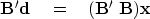(14)
Equation (14) is the classic result of least-squares fitting of data to a collection of regressors. Obviously, the same matrix form applies when there are more than two regressors and each vector has more than three components. Equation (14) leads to an analytic solution forusing an inverse matrix. To solve formally for the unknown,we premultiply by the inverse matrix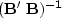: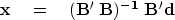(15)
Equation (15) is the central result of least-squares analysis. We see it everywhere.

Equation (12) is an example of what is called a covariance matrix.'' Such matrices usually need to be inverted, and in equation (15) you already see an example of the occurrence of an inverse covariance matrix. Any description of an application of least-squares fitting will generally include some discussion of the covariance matrix--how it will be computed, assumed, or estimated, and how its inverse will be found or approximated. In chapterwe found the need to weight residuals by the inverse of their scale. That was our first example of the occurrence of an inverse covariance matrix--although in that case the matrix size was only.

In our first manipulation of matrix algebra, we move around some parentheses in (14):(16)
Moving the parentheses implies a regrouping of terms or a reordering of a computation. You can verify the validity of moving the parentheses by writing (16) in full as the set of two equations it represents. Equation (14) led to the analytic'' solution (15). In a later section on conjugate gradients, we will see that equation (16) expresses better than (15) the philosophy of computation.

Notice how equation (16) invites us to cancel the matrixfrom each side. We cannot do that of course, becauseis not a number, nor is it a square matrix with an inverse. If you really want to cancel the matrix, you may, but the equation is then only an approximation that restates our original goal (1):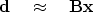(17)

A speedy problem solver might ignore the mathematics covering the previous page, study his or her application until he or she is able to write the statement of wishes (17) = (1), premultiply by,replaceby =, getting (14), and take (14) to a simultaneous equation-solving program to get.

The formal literature does not speak of statement of wishes'' but of regression," which is the same concept. In a regression, there is an abstract vector called the residual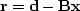whose components should all be small. Formally this is often written as: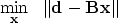(18)
The notation above with two pairs of vertical lines looks like double absolute value, but we can understand it as a reminder to square and sum all the components. This notation is more explicit about what is being minimized, but I often find myself sketching out applications in the form of a statement of wishes,'' which I call a regression.''Next: Inverse filter example Up: Model fitting by least Previous: Model fitting by least
Stanford Exploration Project
10/21/1998